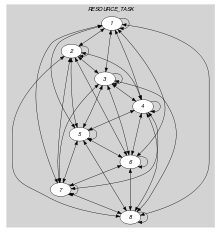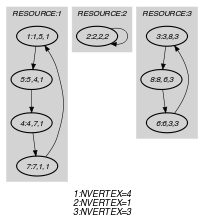## 5.106. cycle_resource

Origin

CHIP

Constraint

$\mathrm{𝚌𝚢𝚌𝚕𝚎}_\mathrm{𝚛𝚎𝚜𝚘𝚞𝚛𝚌𝚎}\left(\mathrm{𝚁𝙴𝚂𝙾𝚄𝚁𝙲𝙴},\mathrm{𝚃𝙰𝚂𝙺}\right)$

Arguments
 $\mathrm{𝚁𝙴𝚂𝙾𝚄𝚁𝙲𝙴}$ $\mathrm{𝚌𝚘𝚕𝚕𝚎𝚌𝚝𝚒𝚘𝚗}\left(\mathrm{𝚒𝚍}-\mathrm{𝚒𝚗𝚝},\mathrm{𝚏𝚒𝚛𝚜𝚝}_\mathrm{𝚝𝚊𝚜𝚔}-\mathrm{𝚍𝚟𝚊𝚛},\mathrm{𝚗𝚋}_\mathrm{𝚝𝚊𝚜𝚔}-\mathrm{𝚍𝚟𝚊𝚛}\right)$ $\mathrm{𝚃𝙰𝚂𝙺}$ $\mathrm{𝚌𝚘𝚕𝚕𝚎𝚌𝚝𝚒𝚘𝚗}\left(\mathrm{𝚒𝚍}-\mathrm{𝚒𝚗𝚝},\mathrm{𝚗𝚎𝚡𝚝}_\mathrm{𝚝𝚊𝚜𝚔}-\mathrm{𝚍𝚟𝚊𝚛},\mathrm{𝚛𝚎𝚜𝚘𝚞𝚛𝚌𝚎}-\mathrm{𝚍𝚟𝚊𝚛}\right)$
Restrictions
 $\mathrm{𝚛𝚎𝚚𝚞𝚒𝚛𝚎𝚍}$$\left(\mathrm{𝚁𝙴𝚂𝙾𝚄𝚁𝙲𝙴},\left[\mathrm{𝚒𝚍},\mathrm{𝚏𝚒𝚛𝚜𝚝}_\mathrm{𝚝𝚊𝚜𝚔},\mathrm{𝚗𝚋}_\mathrm{𝚝𝚊𝚜𝚔}\right]\right)$ $\mathrm{𝚁𝙴𝚂𝙾𝚄𝚁𝙲𝙴}.\mathrm{𝚒𝚍}\ge 1$ $\mathrm{𝚁𝙴𝚂𝙾𝚄𝚁𝙲𝙴}.\mathrm{𝚒𝚍}\le |\mathrm{𝚁𝙴𝚂𝙾𝚄𝚁𝙲𝙴}|$ $\mathrm{𝚍𝚒𝚜𝚝𝚒𝚗𝚌𝚝}$$\left(\mathrm{𝚁𝙴𝚂𝙾𝚄𝚁𝙲𝙴},\mathrm{𝚒𝚍}\right)$ $\mathrm{𝚁𝙴𝚂𝙾𝚄𝚁𝙲𝙴}.\mathrm{𝚏𝚒𝚛𝚜𝚝}_\mathrm{𝚝𝚊𝚜𝚔}\ge 1$ $\mathrm{𝚁𝙴𝚂𝙾𝚄𝚁𝙲𝙴}.\mathrm{𝚏𝚒𝚛𝚜𝚝}_\mathrm{𝚝𝚊𝚜𝚔}\le |\mathrm{𝚁𝙴𝚂𝙾𝚄𝚁𝙲𝙴}|+|\mathrm{𝚃𝙰𝚂𝙺}|$ $\mathrm{𝚁𝙴𝚂𝙾𝚄𝚁𝙲𝙴}.\mathrm{𝚗𝚋}_\mathrm{𝚝𝚊𝚜𝚔}\ge 0$ $\mathrm{𝚁𝙴𝚂𝙾𝚄𝚁𝙲𝙴}.\mathrm{𝚗𝚋}_\mathrm{𝚝𝚊𝚜𝚔}\le |\mathrm{𝚃𝙰𝚂𝙺}|$ $\mathrm{𝚛𝚎𝚚𝚞𝚒𝚛𝚎𝚍}$$\left(\mathrm{𝚃𝙰𝚂𝙺},\left[\mathrm{𝚒𝚍},\mathrm{𝚗𝚎𝚡𝚝}_\mathrm{𝚝𝚊𝚜𝚔},\mathrm{𝚛𝚎𝚜𝚘𝚞𝚛𝚌𝚎}\right]\right)$ $\mathrm{𝚃𝙰𝚂𝙺}.\mathrm{𝚒𝚍}>|\mathrm{𝚁𝙴𝚂𝙾𝚄𝚁𝙲𝙴}|$ $\mathrm{𝚃𝙰𝚂𝙺}.\mathrm{𝚒𝚍}\le |\mathrm{𝚁𝙴𝚂𝙾𝚄𝚁𝙲𝙴}|+|\mathrm{𝚃𝙰𝚂𝙺}|$ $\mathrm{𝚍𝚒𝚜𝚝𝚒𝚗𝚌𝚝}$$\left(\mathrm{𝚃𝙰𝚂𝙺},\mathrm{𝚒𝚍}\right)$ $\mathrm{𝚃𝙰𝚂𝙺}.\mathrm{𝚗𝚎𝚡𝚝}_\mathrm{𝚝𝚊𝚜𝚔}\ge 1$ $\mathrm{𝚃𝙰𝚂𝙺}.\mathrm{𝚗𝚎𝚡𝚝}_\mathrm{𝚝𝚊𝚜𝚔}\le |\mathrm{𝚁𝙴𝚂𝙾𝚄𝚁𝙲𝙴}|+|\mathrm{𝚃𝙰𝚂𝙺}|$ $\mathrm{𝚃𝙰𝚂𝙺}.\mathrm{𝚛𝚎𝚜𝚘𝚞𝚛𝚌𝚎}\ge 1$ $\mathrm{𝚃𝙰𝚂𝙺}.\mathrm{𝚛𝚎𝚜𝚘𝚞𝚛𝚌𝚎}\le |\mathrm{𝚁𝙴𝚂𝙾𝚄𝚁𝙲𝙴}|$
Purpose

Consider a digraph $G$ defined as follows:

• To each item of the $\mathrm{𝚁𝙴𝚂𝙾𝚄𝚁𝙲𝙴}$ and $\mathrm{𝚃𝙰𝚂𝙺}$ collections corresponds one vertex of $G$. A vertex that was generated from an item of the $\mathrm{𝚁𝙴𝚂𝙾𝚄𝚁𝙲𝙴}$ (respectively $\mathrm{𝚃𝙰𝚂𝙺}$) collection is called a resource vertex (respectively task vertex).

• There is an arc from a resource vertex $r$ to a task vertex $t$ if $t\in \mathrm{𝚁𝙴𝚂𝙾𝚄𝚁𝙲𝙴}\left[r\right].\mathrm{𝚏𝚒𝚛𝚜𝚝}_\mathrm{𝚝𝚊𝚜𝚔}$.

• There is an arc from a task vertex $t$ to a resource vertex $r$ if $r\in \mathrm{𝚃𝙰𝚂𝙺}\left[t\right].\mathrm{𝚗𝚎𝚡𝚝}_\mathrm{𝚝𝚊𝚜𝚔}$.

• There is an arc from a task vertex ${t}_{1}$ to a task vertex ${t}_{2}$ if ${t}_{2}\in \mathrm{𝚃𝙰𝚂𝙺}\left[{t}_{1}\right].\mathrm{𝚗𝚎𝚡𝚝}_\mathrm{𝚝𝚊𝚜𝚔}$.

• There is no arc between two resource vertices.

Enforce to cover $G$ in such a way that each vertex belongs to a single circuit. Each circuit is made up from a single resource vertex and zero, one or more task vertices. For each resource-vertex a domain variable indicates how many task-vertices belong to the corresponding circuit. For each task a domain variable provides the identifier of the resource that can effectively handle that task.

Example
$\left(\begin{array}{c}〈\begin{array}{ccc}\mathrm{𝚒𝚍}-1\hfill & \mathrm{𝚏𝚒𝚛𝚜𝚝}_\mathrm{𝚝𝚊𝚜𝚔}-5\hfill & \mathrm{𝚗𝚋}_\mathrm{𝚝𝚊𝚜𝚔}-3,\hfill \\ \mathrm{𝚒𝚍}-2\hfill & \mathrm{𝚏𝚒𝚛𝚜𝚝}_\mathrm{𝚝𝚊𝚜𝚔}-2\hfill & \mathrm{𝚗𝚋}_\mathrm{𝚝𝚊𝚜𝚔}-0,\hfill \\ \mathrm{𝚒𝚍}-3\hfill & \mathrm{𝚏𝚒𝚛𝚜𝚝}_\mathrm{𝚝𝚊𝚜𝚔}-8\hfill & \mathrm{𝚗𝚋}_\mathrm{𝚝𝚊𝚜𝚔}-2\hfill \end{array}〉,\hfill \\ 〈\begin{array}{ccc}\mathrm{𝚒𝚍}-4\hfill & \mathrm{𝚗𝚎𝚡𝚝}_\mathrm{𝚝𝚊𝚜𝚔}-7\hfill & \mathrm{𝚛𝚎𝚜𝚘𝚞𝚛𝚌𝚎}-1,\hfill \\ \mathrm{𝚒𝚍}-5\hfill & \mathrm{𝚗𝚎𝚡𝚝}_\mathrm{𝚝𝚊𝚜𝚔}-4\hfill & \mathrm{𝚛𝚎𝚜𝚘𝚞𝚛𝚌𝚎}-1,\hfill \\ \mathrm{𝚒𝚍}-6\hfill & \mathrm{𝚗𝚎𝚡𝚝}_\mathrm{𝚝𝚊𝚜𝚔}-3\hfill & \mathrm{𝚛𝚎𝚜𝚘𝚞𝚛𝚌𝚎}-3,\hfill \\ \mathrm{𝚒𝚍}-7\hfill & \mathrm{𝚗𝚎𝚡𝚝}_\mathrm{𝚝𝚊𝚜𝚔}-1\hfill & \mathrm{𝚛𝚎𝚜𝚘𝚞𝚛𝚌𝚎}-1,\hfill \\ \mathrm{𝚒𝚍}-8\hfill & \mathrm{𝚗𝚎𝚡𝚝}_\mathrm{𝚝𝚊𝚜𝚔}-6\hfill & \mathrm{𝚛𝚎𝚜𝚘𝚞𝚛𝚌𝚎}-3\hfill \end{array}〉\hfill \end{array}\right)$

The $\mathrm{𝚌𝚢𝚌𝚕𝚎}_\mathrm{𝚛𝚎𝚜𝚘𝚞𝚛𝚌𝚎}$ constraint holds since the graph corresponding to the vertices described by its arguments consists of the following 3 disjoint circuits:

• The first circuit involves the resource vertex 1 as well as the task vertices 5, 4 and 7.

• The second circuit is limited to the resource vertex 2.

• Finally the third circuit is made up from the remaining vertices, namely the resource vertex 3 and the task vertices 8 and 6.

Typical
 $|\mathrm{𝚁𝙴𝚂𝙾𝚄𝚁𝙲𝙴}|>1$ $|\mathrm{𝚃𝙰𝚂𝙺}|>1$ $|\mathrm{𝚃𝙰𝚂𝙺}|>|\mathrm{𝚁𝙴𝚂𝙾𝚄𝚁𝙲𝙴}|$
Symmetries
• Items of $\mathrm{𝚁𝙴𝚂𝙾𝚄𝚁𝙲𝙴}$ are permutable.

• Items of $\mathrm{𝚃𝙰𝚂𝙺}$ are permutable.

• All occurrences of two distinct values in $\mathrm{𝚁𝙴𝚂𝙾𝚄𝚁𝙲𝙴}.\mathrm{𝚒𝚍}$ or $\mathrm{𝚃𝙰𝚂𝙺}.\mathrm{𝚛𝚎𝚜𝚘𝚞𝚛𝚌𝚎}$ can be swapped.

Usage

This constraint is useful for some vehicles routing problem where the number of locations to visit depends of the vehicle type that is actually used. The resource attribute allows expressing various constraints such as:

• The compatibility or incompatibility between tasks and vehicles,

• The fact that certain tasks should be performed by the same vehicle,

• The pre-assignment of certain tasks to a given vehicle.

Remark

This constraint could be expressed with the $\mathrm{𝚌𝚢𝚌𝚕𝚎}$ constraint of CHIP by using the following optional parameters:

Keywords
Derived Collection
$\mathrm{𝚌𝚘𝚕}\left(\begin{array}{c}\mathrm{𝚁𝙴𝚂𝙾𝚄𝚁𝙲𝙴}_\mathrm{𝚃𝙰𝚂𝙺}-\mathrm{𝚌𝚘𝚕𝚕𝚎𝚌𝚝𝚒𝚘𝚗}\left(\begin{array}{c}\mathrm{𝚒𝚗𝚍𝚎𝚡}-\mathrm{𝚒𝚗𝚝},\hfill \\ \mathrm{𝚜𝚞𝚌𝚌}-\mathrm{𝚍𝚟𝚊𝚛},\hfill \\ \mathrm{𝚗𝚊𝚖𝚎}-\mathrm{𝚍𝚟𝚊𝚛}\hfill \end{array}\right),\hfill \\ \left[\begin{array}{c}\mathrm{𝚒𝚝𝚎𝚖}\left(\begin{array}{c}\mathrm{𝚒𝚗𝚍𝚎𝚡}-\mathrm{𝚁𝙴𝚂𝙾𝚄𝚁𝙲𝙴}.\mathrm{𝚒𝚍},\hfill \\ \mathrm{𝚜𝚞𝚌𝚌}-\mathrm{𝚁𝙴𝚂𝙾𝚄𝚁𝙲𝙴}.\mathrm{𝚏𝚒𝚛𝚜𝚝}_\mathrm{𝚝𝚊𝚜𝚔},\hfill \\ \mathrm{𝚗𝚊𝚖𝚎}-\mathrm{𝚁𝙴𝚂𝙾𝚄𝚁𝙲𝙴}.\mathrm{𝚒𝚍}\hfill \end{array}\right),\hfill \\ \mathrm{𝚒𝚝𝚎𝚖}\left(\begin{array}{c}\mathrm{𝚒𝚗𝚍𝚎𝚡}-\mathrm{𝚃𝙰𝚂𝙺}.\mathrm{𝚒𝚍},\hfill \\ \mathrm{𝚜𝚞𝚌𝚌}-\mathrm{𝚃𝙰𝚂𝙺}.\mathrm{𝚗𝚎𝚡𝚝}_\mathrm{𝚝𝚊𝚜𝚔},\hfill \\ \mathrm{𝚗𝚊𝚖𝚎}-\mathrm{𝚃𝙰𝚂𝙺}.\mathrm{𝚛𝚎𝚜𝚘𝚞𝚛𝚌𝚎}\hfill \end{array}\right)\hfill \end{array}\right]\hfill \end{array}\right)$
Arc input(s)

$\mathrm{𝚁𝙴𝚂𝙾𝚄𝚁𝙲𝙴}_\mathrm{𝚃𝙰𝚂𝙺}$

Arc generator
$\mathrm{𝐶𝐿𝐼𝑄𝑈𝐸}$$↦\mathrm{𝚌𝚘𝚕𝚕𝚎𝚌𝚝𝚒𝚘𝚗}\left(\mathrm{𝚛𝚎𝚜𝚘𝚞𝚛𝚌𝚎}_\mathrm{𝚝𝚊𝚜𝚔}\mathtt{1},\mathrm{𝚛𝚎𝚜𝚘𝚞𝚛𝚌𝚎}_\mathrm{𝚝𝚊𝚜𝚔}\mathtt{2}\right)$

Arc arity
Arc constraint(s)
 $•\mathrm{𝚛𝚎𝚜𝚘𝚞𝚛𝚌𝚎}_\mathrm{𝚝𝚊𝚜𝚔}\mathtt{1}.\mathrm{𝚜𝚞𝚌𝚌}=\mathrm{𝚛𝚎𝚜𝚘𝚞𝚛𝚌𝚎}_\mathrm{𝚝𝚊𝚜𝚔}\mathtt{2}.\mathrm{𝚒𝚗𝚍𝚎𝚡}$ $•\mathrm{𝚛𝚎𝚜𝚘𝚞𝚛𝚌𝚎}_\mathrm{𝚝𝚊𝚜𝚔}\mathtt{1}.\mathrm{𝚗𝚊𝚖𝚎}=\mathrm{𝚛𝚎𝚜𝚘𝚞𝚛𝚌𝚎}_\mathrm{𝚝𝚊𝚜𝚔}\mathtt{2}.\mathrm{𝚗𝚊𝚖𝚎}$
Graph property(ies)
 $•$$\mathrm{𝐍𝐓𝐑𝐄𝐄}$$=0$ $•$$\mathrm{𝐍𝐂𝐂}$$=|\mathrm{𝚁𝙴𝚂𝙾𝚄𝚁𝙲𝙴}|$ $•$$\mathrm{𝐍𝐕𝐄𝐑𝐓𝐄𝐗}$$=|\mathrm{𝚁𝙴𝚂𝙾𝚄𝚁𝙲𝙴}|+|\mathrm{𝚃𝙰𝚂𝙺}|$

Graph class
$\mathrm{𝙾𝙽𝙴}_\mathrm{𝚂𝚄𝙲𝙲}$

For all items of $\mathrm{𝚁𝙴𝚂𝙾𝚄𝚁𝙲𝙴}$:

Arc input(s)

$\mathrm{𝚁𝙴𝚂𝙾𝚄𝚁𝙲𝙴}_\mathrm{𝚃𝙰𝚂𝙺}$

Arc generator
$\mathrm{𝐶𝐿𝐼𝑄𝑈𝐸}$$↦\mathrm{𝚌𝚘𝚕𝚕𝚎𝚌𝚝𝚒𝚘𝚗}\left(\mathrm{𝚛𝚎𝚜𝚘𝚞𝚛𝚌𝚎}_\mathrm{𝚝𝚊𝚜𝚔}\mathtt{1},\mathrm{𝚛𝚎𝚜𝚘𝚞𝚛𝚌𝚎}_\mathrm{𝚝𝚊𝚜𝚔}\mathtt{2}\right)$

Arc arity
Arc constraint(s)
 $•\mathrm{𝚛𝚎𝚜𝚘𝚞𝚛𝚌𝚎}_\mathrm{𝚝𝚊𝚜𝚔}\mathtt{1}.\mathrm{𝚜𝚞𝚌𝚌}=\mathrm{𝚛𝚎𝚜𝚘𝚞𝚛𝚌𝚎}_\mathrm{𝚝𝚊𝚜𝚔}\mathtt{2}.\mathrm{𝚒𝚗𝚍𝚎𝚡}$ $•\mathrm{𝚛𝚎𝚜𝚘𝚞𝚛𝚌𝚎}_\mathrm{𝚝𝚊𝚜𝚔}\mathtt{1}.\mathrm{𝚗𝚊𝚖𝚎}=\mathrm{𝚛𝚎𝚜𝚘𝚞𝚛𝚌𝚎}_\mathrm{𝚝𝚊𝚜𝚔}\mathtt{2}.\mathrm{𝚗𝚊𝚖𝚎}$ $•\mathrm{𝚛𝚎𝚜𝚘𝚞𝚛𝚌𝚎}_\mathrm{𝚝𝚊𝚜𝚔}\mathtt{1}.\mathrm{𝚗𝚊𝚖𝚎}=\mathrm{𝚁𝙴𝚂𝙾𝚄𝚁𝙲𝙴}.\mathrm{𝚒𝚍}$
Graph property(ies)
$\mathrm{𝐍𝐕𝐄𝐑𝐓𝐄𝐗}$$=\mathrm{𝚁𝙴𝚂𝙾𝚄𝚁𝙲𝙴}.\mathrm{𝚗𝚋}_\mathrm{𝚝𝚊𝚜𝚔}+1$

Graph model

The graph model of the $\mathrm{𝚌𝚢𝚌𝚕𝚎}_\mathrm{𝚛𝚎𝚜𝚘𝚞𝚛𝚌𝚎}$ constraint illustrates the following points:

• How to differentiate the constraint on the length of a circuit according to a resource that is assigned to a circuit? This is achieved by introducing a collection of resources and by asking a different graph property for each item of that collection.

• How to introduce the concept of name that corresponds to the resource that handles a given task? This is done by adding to the arc constraint associated with the $\mathrm{𝚌𝚢𝚌𝚕𝚎}$ constraint the condition that the name variables of two consecutive vertices should be equal.

Part (A) of Figure 5.106.1 shows the initial graphs (of the second graph constraint) associated with resources 1, 2 and 3 of the Example slot. Part (B) of Figure 5.106.1 shows the corresponding final graphs (of the second graph constraint) associated with resources 1, 2 and 3. Since we use the $\mathrm{𝐍𝐕𝐄𝐑𝐓𝐄𝐗}$ graph property, the vertices of the final graphs are stressed in bold. To each resource corresponds a circuit of respectively 3, 0 and 2 task-vertices.

##### Figure 5.106.1. Initial and final graph of the $\mathrm{𝚌𝚢𝚌𝚕𝚎}_\mathrm{𝚛𝚎𝚜𝚘𝚞𝚛𝚌𝚎}$ constraint(a) (b)
Signature

Since the initial graph of the first graph constraint contains $|\mathrm{𝚁𝙴𝚂𝙾𝚄𝚁𝙲𝙴}|+|\mathrm{𝚃𝙰𝚂𝙺}|$ vertices, the corresponding final graph cannot have more than $|\mathrm{𝚁𝙴𝚂𝙾𝚄𝚁𝙲𝙴}|+|\mathrm{𝚃𝙰𝚂𝙺}|$ vertices. Therefore we can rewrite the graph property $\mathrm{𝐍𝐕𝐄𝐑𝐓𝐄𝐗}$ $=$ $|\mathrm{𝚁𝙴𝚂𝙾𝚄𝚁𝙲𝙴}|+|\mathrm{𝚃𝙰𝚂𝙺}|$ to $\mathrm{𝐍𝐕𝐄𝐑𝐓𝐄𝐗}$ $\ge$ $|\mathrm{𝚁𝙴𝚂𝙾𝚄𝚁𝙲𝙴}|+|\mathrm{𝚃𝙰𝚂𝙺}|$ and simplify $\underline{\overline{\mathrm{𝐍𝐕𝐄𝐑𝐓𝐄𝐗}}}$ to $\overline{\mathrm{𝐍𝐕𝐄𝐑𝐓𝐄𝐗}}$.